# Appendix

##### A Proof of Proposition 1

Without loss of generality, let X = {x1,x2,,xl} and Y = {y1,y2,,yl} with d(xi,yi) ε for i = 1, 2,,l. Let Zi be a binary random variable such that Zi= 1 if both xi and yi are chosen as sampled frames, and 0 otherwise. Since Wm is the total number of similar pairs between the two sets of sampled frames, it can be computed by summing all the Zi's: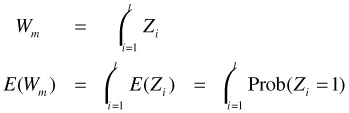Since we independently sample m frames from each sequence, the probability that Zi = 1 for any i is (m/l)2. This implies that E(W) = m2/l.

##### B Proof of Proposition 2

To simplify the notation, let ρ(X,Y) = vvs(X,Y;ε) and(X,Y) = vssb(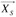,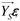, m). For an arbitrary pair of X and Y, we can bound the probability of the event |ρ(X,Y)-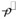(X,Y)|by the Hoeffding Inequality :

 (28.19)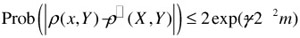To find an upper bound for Perr(m), we can combine (0.1) and the union bound as follows: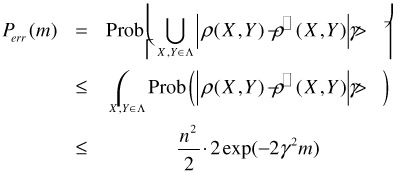A sufficient condition for Perr(m) δ is thus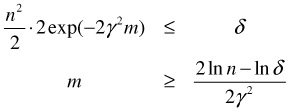##### C Proof of Proposition 3

For each term inside the summation on the right hand side of Equation (28.12), d(x, y) must be smaller than or equal to ε. If d(x, y) ε, our assumption implies that both x and y must be in the same cluster C belonging to both [X]ε and [Y]ε. As a result, we can rewrite the right hand side of Equation (28.12) based only on clusters in [X]ε [Y]ε:

 (28.20)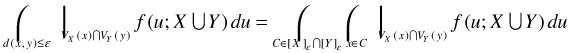Based on the definition of a Voronoi Cell, it is easy to see that VX(z)VY(z) = VXY(z) for all z C with C [X]ε[Y]ε. Substituting this relationship into Equation (28.12), we obtain: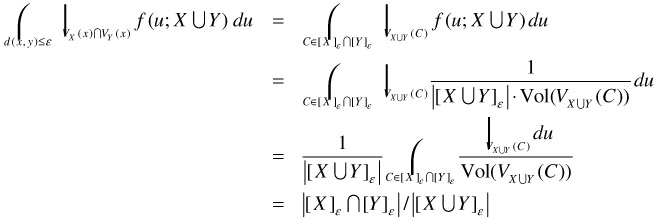Finally, we note that [X]ε[Y]ε is in fact the set of all Similar Clusters in [XY]ε, and thus the last expression equals to the IVS. The reason is that for any Similar Cluster C in [XY]ε, C must have at least one x X and one y Y such that d(x, y) ε. By our assumption, C must be in both [X]ε and [Y]ε.

##### D Proof of Proposition 4

Without loss of generality, we assume that x1 is at the origin with all zeros, and x2 has k 1's in the rightmost positions. Clearly, d(x1,x2) = k. Throughout this proof, when we mention a particular sequence Y Γ, we adopt the convention that Y = {y1, y2} with d(x1,y1) ε and d(x2,y2) ≤ε.

We first divide the region A into two partitions based on the proximity to the frames in X:

A1 := {s A: gX(s) = x1} and A2 := {s A: gX(s) = x2}

We adopt the convention that if there are multiple frames in a video Z that are equidistant to a random vector s, gZ(s) is defined to be the frame furthest away from the origin. This implies that all vectors equidistant to both frames in X are elements of A2. Let s be an arbitrary vector in H, and R be the random variable that denotes the number of 1's in the rightmost k bit positions of s. The probability that R equals to a particular r with r k is as follows: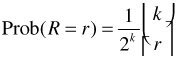Thus, R follows a binomial distribution of parameters k and 1/2. In this proof, we show the following relationship between A2 and R:

 (28.21)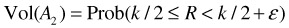With an almost identical argument, we can show the following:

 (28.22)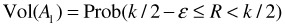Since Vol(A) = Vol(A1) + Vol(A2), the desired result follows.

To prove Equation (28.21), we first show if k/2R<k/2+ε, then s A2. Assuming the definitions for A and A2, we need to show two things: (1) gX(s) = x2; (2) there exists a Y Γ such that s G(X,Y;ε), or equivalently, gY(s) = y1. To show (1), we rewrite R = k/2 + N where 0N<ε and let the number of 1's in s be L. Consider the distances between s and x1, and between s and x2. Since x1 is all zeros, d(s, x,) = L. As x2 has all its 1's in the rightmost k position, d(s,x2) = (L-R) + (k-R) = L + k-2R. Thus,

 d(s,x1)-d(s,x2) = L-L(+k-2R) = 2R-k = 2N≥0,

which implies that gX(s) = x2. To show (2), we define y1 to be a h -bit binary number with all zeros, except for ε 1's in the positions which are randomly chosen from the R 1's in the rightmost k bits of s. We can do that because Rk/2≥ε . Clearly, d(x1,y1) = ε and d(s,y1) = L-ε. Next, we define y2 by toggling ε out of k 1's in x2. The positions we toggle are randomly chosen from the same R 1's bits in s. As a result, d(x2, y2) = ε and d(s, y2) = (L-R) + (k-R + ε) = L + ε-2N. Clearly, Y:={y1,y2} belongs to Γ. Since

 d(s,y2)-d(s,y1) = (L + ε-2N)-(L-ε) = 2(ε-N)>0

gY(s) = y1 and, consequently, s G(X,Y;ε).

Now we show the other direction: if s A2, then k/2 R<k/2+ ε. Since s A2, we have gX(s) = x2 which implies that L = d(s,x1)d(s,x2) = L + k-2R or k/2R. Also, there exists a Y Γ with s G(X, Y; ε). This implies gY(s) = y2, or equivalently, d(s,yl)<d(s, y2). This inequality is strict as equality will force gY(s) = y2 by the convention we adopt for gY( ). The terms on both sides of the inequality can be bounded using the triangle inequality: d(s,y1)d(s,x1)-d(x1,y1) = L-ε and d(s, y2)d(s, x2) + d(x2,y2) = L + k-2R + ε. Combining both bounds, we have

L-ε<L + k-2R + ε R<k/2 + ε

This completes the proof for Equation (28.21). The proof of Equation (28.22) follows the same argument with the roles of x1 and x2 reversed. Combining the two equations, we obtain the desired result.

##### E Proof of Proposition 5

We prove the case for video X and the proof is identical for Y. Since s G(X,Y;ε), we have d(gX(s),gY(s)) > ε and there exists x X with d(x,gY(s))≤ε. Since all Similar Clusters in [XY]ε are ε-compact, gX(s) cannot be in the same cluster with x and gY(s). Thus, we have d(gX(s), x) > ε. It remains to show that d(x,s)-d(gX(s),s) 2ε. Using the triangle inequality, we have

 (28.23)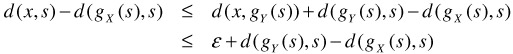s G(X,Y;ε) also implies that there exists y Y such that d(y, gY (s)) ε. By the definition of gY (s), d(gY (s), s) d(y,s). Thus, we can replace gY (s) with y in Equation (28.23) and combine with the triangle inequality to obtain:

 d(x,s)-d(gX(s),s) ≤ ε + d(y,s)-d(gX(s),s) ≤ ε + d(y,gX(s)) ≤ 2ε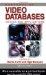Handbook of Video Databases: Design and Applications (Internet and Communications)
ISBN: 084937006X
EAN: 2147483647
Year: 2003
Pages: 393